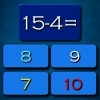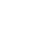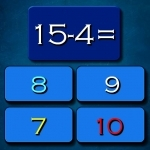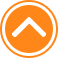×

PlaySubtraction Math Challenge71%Device: 💻 Pc   📱 Mobile
Rating: 71%
🎲Subtraction Math Challenge

Subtraction Math Challenge is fun math game suitable for all ages. I hope you like math and this game is just to test your math skills. Ten seconds to answer each quastion. Have fun playing. Welcome to play Subtraction Math Challenge game for free in friv90000.org. Subtraction Math Challenge is a wonderful game from our great collection of Friv 90000 games which are updated everytime.

🎮 Instructions

Use Left Mouse button to Play

📂 Categories

puzzle, arcade, mathka, numbers, school, thinking, mathematical, mathematics, igrice, mathematic, math..

👌 Recommended
📌 Tags